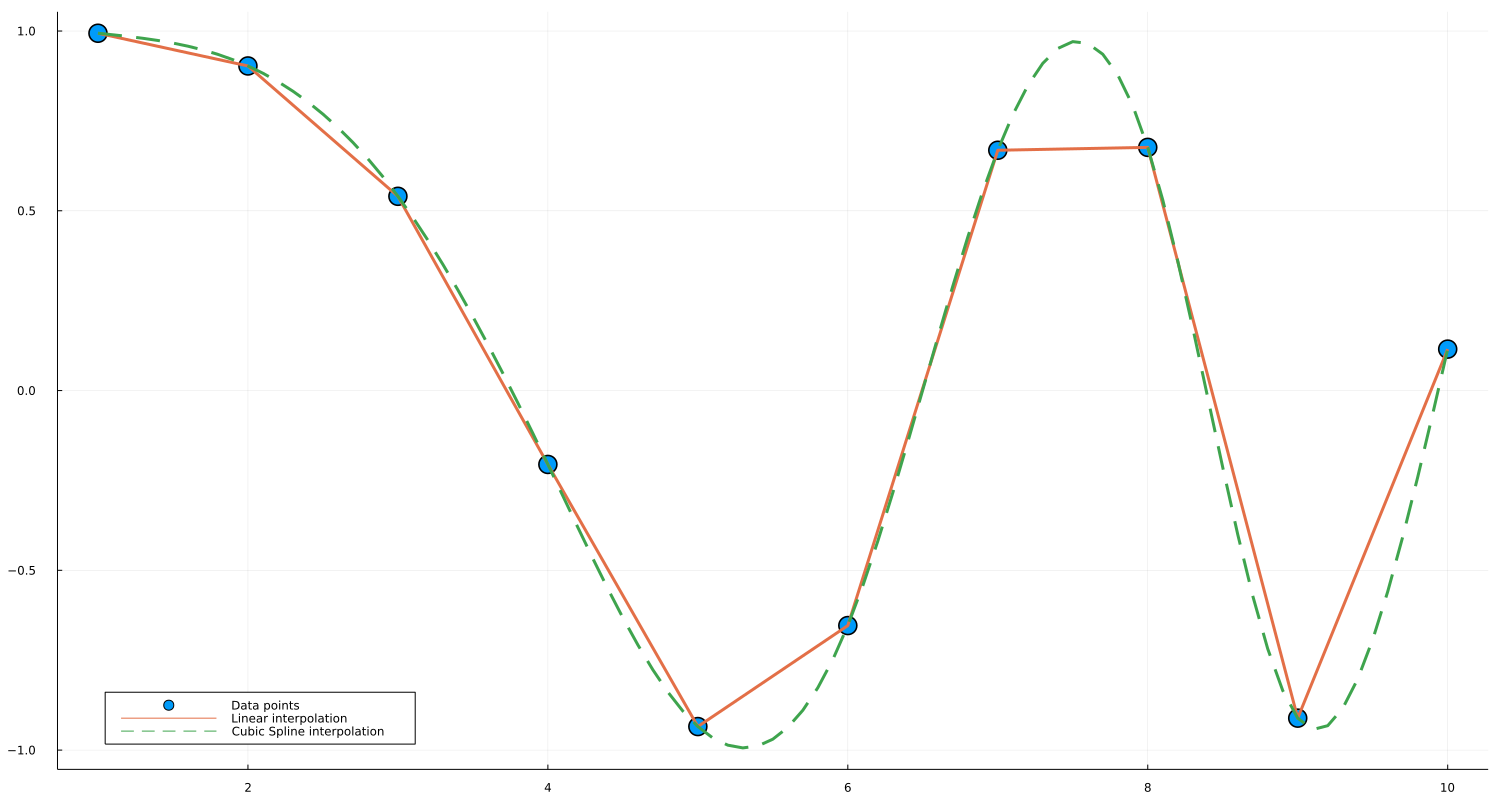## Convenience notation

For constant, linear, and cubic spline interpolations, constant_interpolation, linear_interpolation, and cubic_spline_interpolation can be used to create interpolating and extrapolating objects handily.

### Motivating Example

By using the convenience constructor one can simplify expressions. For example, the creation of an interpolation object

extrap_full = extrapolate(scale(interpolate(A, BSpline(Linear())), xs), Line())

can be written as the more readable

extrap = linear_interpolation(xs, A, extrapolation_bc = Line())

by using the convenience constructor.

### Usage

f(x) = log(x)
xs = 1:0.2:5
A = [f(x) for x in xs]

# linear interpolation
interp_linear = linear_interpolation(xs, A)
interp_linear(3) # exactly log(3)
interp_linear(3.1) # approximately log(3.1)

# cubic spline interpolation
interp_cubic = cubic_spline_interpolation(xs, A)
interp_cubic(3) # exactly log(3)
interp_cubic(3.1) # approximately log(3.1)

which support multidimensional data as well:

f(x,y) = log(x+y)
xs = 1:0.2:5
ys = 2:0.1:5
A = [f(x,y) for x in xs, y in ys]

# linear interpolation
interp_linear = linear_interpolation((xs, ys), A)
interp_linear(3, 2) # exactly log(3 + 2)
interp_linear(3.1, 2.1) # approximately log(3.1 + 2.1)

# cubic spline interpolation
interp_cubic = cubic_spline_interpolation((xs, ys), A)
interp_cubic(3, 2) # exactly log(3 + 2)
interp_cubic(3.1, 2.1) # approximately log(3.1 + 2.1)

For extrapolation, i.e., when interpolation objects are evaluated in coordinates outside the range provided in constructors, the default option for a boundary condition is Throw so that they will return an error. Interested users can specify boundary conditions by providing an extra parameter for extrapolation_bc:

f(x) = log(x)
xs = 1:0.2:5
A = [f(x) for x in xs]

# extrapolation with linear boundary conditions
extrap = linear_interpolation(xs, A, extrapolation_bc = Line())

@test extrap(1 - 0.2) # ≈ f(1) - (f(1.2) - f(1))
@test extrap(5 + 0.2) # ≈ f(5) + (f(5) - f(4.8))

You can also use a "fill" value, which gets returned whenever you ask for out-of-range values:

extrap = linear_interpolation(xs, A, extrapolation_bc = NaN)
@test isnan(extrap(5.2))

Irregular grids are supported as well; note that presently only constant_interpolation and linear_interpolation supports irregular grids.

xs = [x^2 for x = 1:0.2:5]
A = [f(x) for x in xs]

# linear interpolation
interp_linear = linear_interpolation(xs, A)
interp_linear(1) # exactly log(1)
interp_linear(1.05) # approximately log(1.05)

### Example with Plots.jl

An interpolated object is also easily capable of being plotted with Plots.jl. A simple example is as follows:

using Interpolations, Plots

# Lower and higher bound of interval
a = 1.0
b = 10.0
# Interval definition
x = a:1.0:b
# This can be any sort of array data, as long as
# length(x) == length(y)
y = @. cos(x^2 / 9.0) # Function application by broadcasting
# Interpolations
itp_linear = linear_interpolation(x, y)
itp_cubic = cubic_spline_interpolation(x, y)
# Interpolation functions
f_linear(x) = itp_linear(x)
f_cubic(x) = itp_cubic(x)
# Plots
width, height = 1500, 800 # not strictly necessary
x_new = a:0.1:b # smoother interval, necessary for cubic spline

scatter(x, y, markersize=10,label="Data points")
plot!(f_linear, x_new, w=3,label="Linear interpolation")
plot!(f_cubic, x_new, linestyle=:dash, w=3, label="Cubic Spline interpolation")
plot!(size = (width, height))
plot!(legend = :bottomleft)

And the generated plot is: